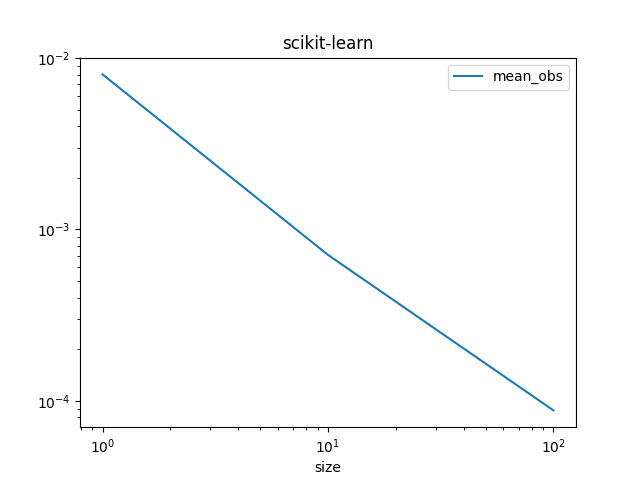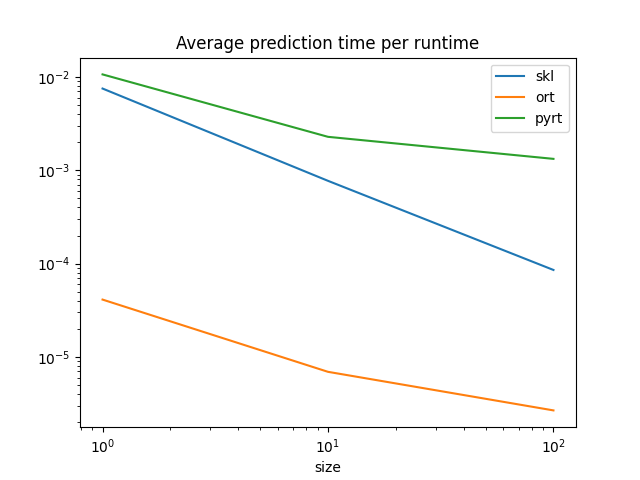# Benchmark ONNX conversion#

Example Train and deploy a scikit-learn pipeline converts a simple model. This example takes a similar example but on random data and compares the processing time required by each option to compute predictions.

## Training a pipeline#

```import numpy
from pandas import DataFrame
from tqdm import tqdm
from sklearn import config_context
from sklearn.datasets import make_regression
from sklearn.ensemble import (
VotingRegressor)
from sklearn.linear_model import LinearRegression
from sklearn.model_selection import train_test_split
from mlprodict.onnxrt import OnnxInference
from onnxruntime import InferenceSession
from skl2onnx import to_onnx
from skl2onnx.tutorial import measure_time

N = 11000
X, y = make_regression(N, n_features=10)
X_train, X_test, y_train, y_test = train_test_split(
X, y, train_size=0.01)
print("Train shape", X_train.shape)
print("Test shape", X_test.shape)

reg2 = RandomForestRegressor(random_state=1)
reg3 = LinearRegression()
ereg = VotingRegressor([('gb', reg1), ('rf', reg2), ('lr', reg3)])
ereg.fit(X_train, y_train)
```
```Train shape (110, 10)
Test shape (10890, 10)
```
```VotingRegressor(estimators=[('gb', GradientBoostingRegressor(random_state=1)),
('rf', RandomForestRegressor(random_state=1)),
('lr', LinearRegression())])```
In a Jupyter environment, please rerun this cell to show the HTML representation or trust the notebook.

## Measure the processing time#

We use function `skl2onnx.tutorial.measure_time()`. The page about assume_finite may be useful if you need to optimize the prediction. We measure the processing time per observation whether or not an observation belongs to a batch or is a single one.

```sizes = [(1, 50), (10, 50), (1000, 10), (10000, 5)]

with config_context(assume_finite=True):
obs = []
for batch_size, repeat in tqdm(sizes):
context = {"ereg": ereg, 'X': X_test[:batch_size]}
mt = measure_time(
"ereg.predict(X)", context, div_by_number=True,
number=10, repeat=repeat)
mt['size'] = context['X'].shape
mt['mean_obs'] = mt['average'] / mt['size']
obs.append(mt)

df_skl = DataFrame(obs)
df_skl
```
```  0%|          | 0/4 [00:00<?, ?it/s]
25%|##5       | 1/4 [00:03<00:10,  3.56s/it]
50%|#####     | 2/4 [00:06<00:06,  3.44s/it]
75%|#######5  | 3/4 [00:10<00:03,  3.58s/it]
100%|##########| 4/4 [00:18<00:00,  5.37s/it]
100%|##########| 4/4 [00:18<00:00,  4.70s/it]
```
average deviation min_exec max_exec repeat number size mean_obs
0 0.007118 0.001354 0.005786 0.012553 50 10 1 0.007118
1 0.006686 0.001005 0.005542 0.010000 50 10 10 0.000669
2 0.037563 0.003221 0.033256 0.045436 10 10 1000 0.000038
3 0.162250 0.008343 0.153190 0.177519 5 10 10000 0.000016

Graphe.

```df_skl.set_index('size')[['mean_obs']].plot(
title="scikit-learn", logx=True, logy=True)
```## ONNX runtime#

The same is done with the two ONNX runtime available.

```onx = to_onnx(ereg, X_train[:1].astype(numpy.float32),
target_opset=14)
sess = InferenceSession(onx.SerializeToString())
oinf = OnnxInference(onx, runtime="python_compiled")

obs = []
for batch_size, repeat in tqdm(sizes):

# scikit-learn
context = {"ereg": ereg, 'X': X_test[:batch_size].astype(numpy.float32)}
mt = measure_time(
"ereg.predict(X)", context, div_by_number=True,
number=10, repeat=repeat)
mt['size'] = context['X'].shape
mt['skl'] = mt['average'] / mt['size']

# onnxruntime
context = {"sess": sess, 'X': X_test[:batch_size].astype(numpy.float32)}
mt2 = measure_time(
"sess.run(None, {'X': X})", context, div_by_number=True,
number=10, repeat=repeat)
mt['ort'] = mt2['average'] / mt['size']

# mlprodict
context = {"oinf": oinf, 'X': X_test[:batch_size].astype(numpy.float32)}
mt2 = measure_time(
"oinf.run({'X': X})['variable']", context, div_by_number=True,
number=10, repeat=repeat)
mt['pyrt'] = mt2['average'] / mt['size']

# end
obs.append(mt)

df = DataFrame(obs)
df
```
```  0%|          | 0/4 [00:00<?, ?it/s]
25%|##5       | 1/4 [00:03<00:10,  3.65s/it]
50%|#####     | 2/4 [00:07<00:07,  3.52s/it]
75%|#######5  | 3/4 [00:18<00:07,  7.29s/it]
100%|##########| 4/4 [00:34<00:00, 10.72s/it]
100%|##########| 4/4 [00:34<00:00,  8.71s/it]
```
average deviation min_exec max_exec repeat number size skl ort pyrt
0 0.007177 0.000710 0.005820 0.010053 50 10 1 0.007177 0.000026 0.000077
1 0.006645 0.000843 0.005670 0.008772 50 10 10 0.000664 0.000010 0.000010
2 0.034182 0.001519 0.032991 0.038628 10 10 1000 0.000034 0.000004 0.000080
3 0.155520 0.003090 0.151416 0.160624 5 10 10000 0.000016 0.000003 0.000014

Graph.

```df.set_index('size')[['skl', 'ort', 'pyrt']].plot(
title="Average prediction time per runtime",
logx=True, logy=True)
```ONNX runtimes are much faster than scikit-learn to predict one observation. scikit-learn is optimized for training, for batch prediction. That explains why scikit-learn and ONNX runtimes seem to converge for big batches. They use similar implementation, parallelization and languages (C++, openmp).

Total running time of the script: ( 0 minutes 55.146 seconds)

Gallery generated by Sphinx-Gallery